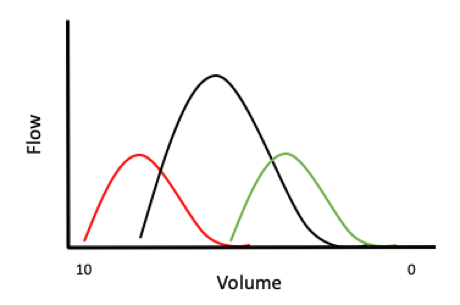🥵 HARD

The chart shown is a simplified volume-flow chart that you could get from the results of spirometry. The black curve is the normal result you would expect, the red and green curves show abnormal pathologies. Do you understand what the results might mean?

Reviewed by: awaiting review###### The above chart shows three volume-flow curves generated by spirometry. The black curve is the normal curve. What type of lung disease would you expect in a person who generated the red (left) curve? (1 mark)

Obstructive lung disease (e.g. chronic asthma, COPD)

###### Why is the maximum flow rate for the red (left) curve not as high as the control? (1 mark)

Airways are narrowed. As flow (Q) is proportionate to radius4, a small narrowing of the airway has a big impact on the volume of air that can be transported per unit time.

###### Why is the lung capacity increased? (1 mark)

You can inspire more than you can expire – during expiration the narrower bronchioles will close sooner so not all inhaled air can be exhaled.

###### What type of lung disease would you expect in a person who generated the green (right) curve? (1 mark)

Restrictive lung disease (e.g. fibrosis)

###### Why is the maximum flow rate lower than the control? (1 mark)

Because the lungs have a smaller capacity, they aren’t able to expand as much. Therefore, there is less elastic energy in the chest wall and lungs so less force to expel the air.

###### How would the functional residual capacity for an obese person compare to that of a normal person? Would the spirometry result for an obese person be more similar to the red (left) or green (right) curve? (3 marks)

Functional residual capacity is the volume of air left in the lungs after normal expiration. The increased weight on an obese person’s chest means they would expel more air, giving a smaller reserve expiratory volume, and it would also slightly reduce the residual capacity, so FRC would be less. This results in a reduced total lung capacity so the flow-volume curve would look more similar to the green (right) curve. Arguably, an obese person’s increased weight adds more resistance to breathing so is similar in some respects to a restrictive lung condition. Scoliosis and hyperkyphosis would have similar effects on lung capacity and shift the curve to the right as well.

###### DLCO (diffusion rate of the lung using carbon monoxide) is a test used to assess the amount of diffusion in the lungs. Compared to average, how would diffusion rates vary for a patient with emphysema and one with fibrosis? (1 mark)

DLCO would be reduced in both. Rate of diffusion is affected by surface area, diffusibility, difference in pressure/concentration and surface thickness:

rate = (area × diffusion coefficient × Δp) / thickness

A patient with emphysema has a reduced number of alveoli therefore they have a reduced surface area. As rate is proportional to surface area, the rate of diffusion is reduced.

In fibrosis, the lining of the lung is thicker. The thicker the membrane, the slower the rate of diffusion.

###### Ignoring other physiological factors, is carbon monoxide or carbon dioxide more likely to diffuse faster across the lung membranes? (1 mark)

Carbon monoxide will diffuse faster because it has a lower molecular weight. Graham’s Law shows how the rate of diffusion of a gas is inversely proportionate to the molecular weight.

###### Using the relationship between flow, velocity and area, explain why the movement of air in the lungs goes from bulk flow to passive diffusion. After which bronchiole division does this occur? (3 marks)

Recall that:

velocity = flow / area

As the bronchioles divide, the total cross-sectional area of the bronchioles increases. The flow will remain largely constant, or reduce due to increased resistance. At some point the cross-sectional area becomes so large, velocity is almost zero/negligible, and diffusion takes over. This occurs around division 16 and is associated with particulates being deposited in this part of the lung.

7 + 1 =